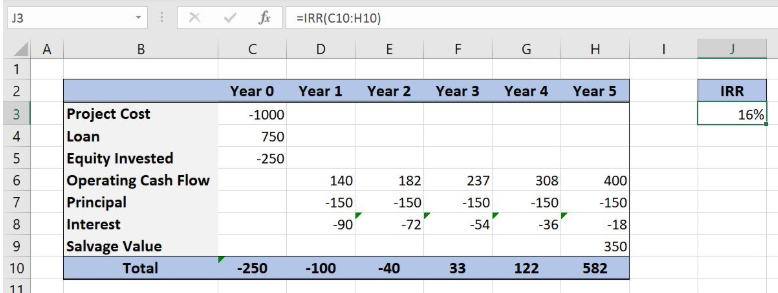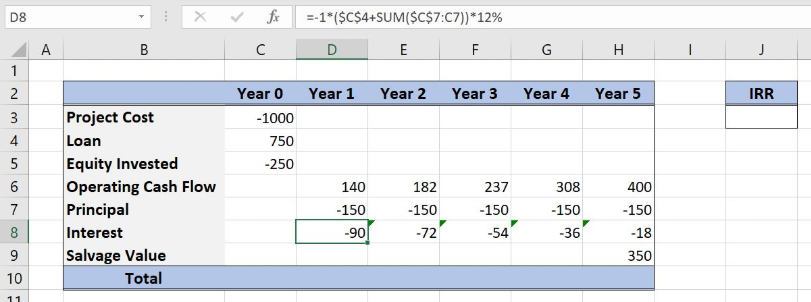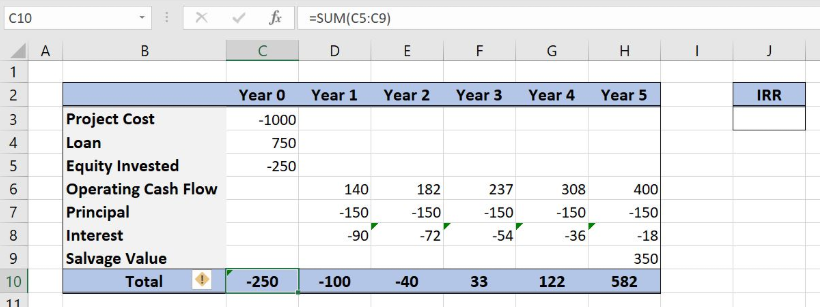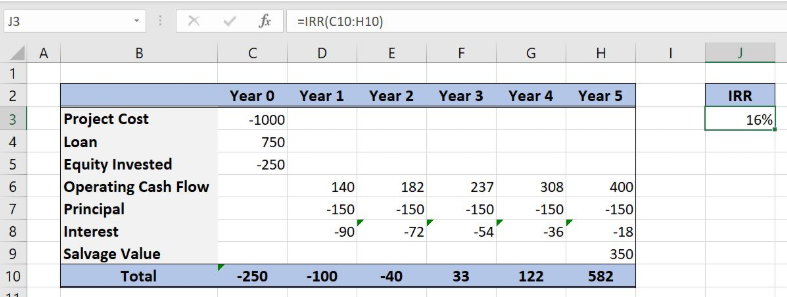Get instant live expert help with Excel or Google Sheets“My Excelchat expert helped me in less than 20 minutes, saving me what would have been 5 hours of work!”

#### Post your problem and you’ll get expert help in seconds.

Your message must be at least 40 characters
Our professional experts are available now. Your privacy is guaranteed.

# Learn How to Find the Equity IRR for a Project in Excel

Excel allows a user to get an internal rate of return of an investment using the IRR function. This step by step tutorial will assist all levels of Excel users in getting an IRR of an investment.Figure 1. The result of the equity IRR function

## Syntax of the SUM Formula

The generic formula for the SUM function is:

`=SUM(number1, [number2], [number3],... )`

The parameters of the SUM function are:

• number1, [number2], [number3],… – numbers that we want to summarize, or a range containing numbers.

## Syntax of the IRR Formula

The generic formula for the IRR function is:

`=IRR (values, [guess])`

The parameters of the IRR function are:

• values – a range of cells containing values, including initial investment and incomes. The investment must have a negative sign, as it is a cost
• [guess] – an estimated value for the expected IRR. This parameter is non-mandatory. If it’s omitted, the function will take a default value of 0.1 (=10%).

## Setting up Our Data for the Equity IRR Function

Let’s look at the structure of the data we will use. In columns C:H, we have years 0-5. In rows, we have incomes and costs per year. In cells C10:H10, we need to get the sum for each year. Finally, in the cell J3, we want to calculate the IRR for the specified cash-flow.

As we can see, Interest in row 8, is calculated based on loan and incomes, using the formula `=-1*(\$C\$4+SUM(\$C\$7:C7))*.12`Figure 2. Data that we will use in the equity IRR example

## Get an IRR of Values Using the IRR Function

In our example, we first want to calculate the sum for all years.

The formula for summarizing looks like:

`=SUM(C5:C9)`

The parameter numbers of the function is the range C5:C9.

To apply the SUM function, we need to follow these steps:

• Select cell C10 and click on it
• Insert the formula:` =SUM(C5:C9)`
• Press enter
• Drag the formula right to the other cells in the row by clicking and dragging the little “+” icon at the bottom-right of the cell.Figure 3. Using the SUM function to sum costs and incomes for every year

Finally, the result in the cell C10 is -250, which is the sum of costs and incomes for the Year 0. Now, we can calculate the IRR using the IRR function and sums for every year.

The formula for IRR looks like:

`=IRR(C10:H10)`

The parameter values of the function is the range C10:H10.

To apply the IRR function, we need to follow these steps:

• Select cell J3 and click on it
• Insert the formula: `=IRR(C10:H10)`
• Press enter.Figure 4. Using the IRR function to calculate the equity IRR

Finally, the internal rate of return in the cell J3 is 16%.

Most of the time, the problem you will need to solve will be more complex than a simple application of a formula or function. If you want to save hours of research and frustration, try our live Excelchat service! Our Excel Experts are available 24/7 to answer any Excel question you may have. We guarantee a connection within 30 seconds and a customized solution within 20 minutes.

Solution examplesI need a formula to pop at the upper left corner of a spreadsheet. If I enter the month "January," I want the column number sum of January =SUM(AB11:AB75) from another section on the same excel page to pop right below the "January" cell, and not display the formula expression, but see the \$100.
Solved by T. Q. in 40 minsCan't add (SUM) in imported numbers from bank account
Solved by F. C. in 40 minsI need a formula to combine D2 to D100 to add together a column of numbers, then take away the same amount on the same row when column E is filled. i.e. column D is a price of an item, so the formula must calculate the total, then when the item is sold an 'a' is marked next to the item in column E, the formula then must deduct this amount from the total
Solved by X. W. in 20 minsI would like to have a diagram in a new sheet, where the horizontal axis is the days, as they are in column DX. Each day shall show the sum of all unique leads of that day, and I would like to be able to check via a box of checkboxes, which facilities are shown, the facilities are in column BC.
Solved by I. A. in 45 minsI am working on a cash flow projection. Part of the projection includes sales commissions. Our sales guys earn a monthly draw and then commission on sales after a certain amount. For example they may earn a monthly salary of 12,500 and earn additional commission after their commission equals \$150,000. What I need excel to do is sum a column if the values in the preceeding columns are greater than \$150,000. I've tried using the sumif and the if function in excel and it's not working correctly either way. Any suggestions?
Solved by G. W. in 19 mins